# Test: Partial Derivatives, Gradient- 2 - Mechanical Engineering

Test Description

## 25 Questions MCQ Test GATE Mechanical (ME) 2023 Mock Test Series - Test: Partial Derivatives, Gradient- 2

Test: Partial Derivatives, Gradient- 2 for Mechanical Engineering 2023 is part of GATE Mechanical (ME) 2023 Mock Test Series preparation. The Test: Partial Derivatives, Gradient- 2 questions and answers have been prepared according to the Mechanical Engineering exam syllabus.The Test: Partial Derivatives, Gradient- 2 MCQs are made for Mechanical Engineering 2023 Exam. Find important definitions, questions, notes, meanings, examples, exercises, MCQs and online tests for Test: Partial Derivatives, Gradient- 2 below.
Solutions of Test: Partial Derivatives, Gradient- 2 questions in English are available as part of our GATE Mechanical (ME) 2023 Mock Test Series for Mechanical Engineering & Test: Partial Derivatives, Gradient- 2 solutions in Hindi for GATE Mechanical (ME) 2023 Mock Test Series course. Download more important topics, notes, lectures and mock test series for Mechanical Engineering Exam by signing up for free. Attempt Test: Partial Derivatives, Gradient- 2 | 25 questions in 75 minutes | Mock test for Mechanical Engineering preparation | Free important questions MCQ to study GATE Mechanical (ME) 2023 Mock Test Series for Mechanical Engineering Exam | Download free PDF with solutions
 1 Crore+ students have signed up on EduRev. Have you?
Test: Partial Derivatives, Gradient- 2 - Question 1

### f(x, y) = x2 + xyz + z Find fx at (1,1,1)

Detailed Solution for Test: Partial Derivatives, Gradient- 2 - Question 1

fx = 2x + yz

Put (x,y,z) = (1,1,1)

fx = 2 + 1 = 3.

Test: Partial Derivatives, Gradient- 2 - Question 2

### Eight people are planning to share equally the cost of a rental car. If one person withdraws from the arrangement and the others share equally the entire cost of the car, then the share of each of the remaining persons increased by:

Detailed Solution for Test: Partial Derivatives, Gradient- 2 - Question 2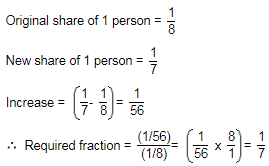Test: Partial Derivatives, Gradient- 2 - Question 3

### The minimum point of the function f(x) = (x2/3) – x is at

Detailed Solution for Test: Partial Derivatives, Gradient- 2 - Question 3

Explanation : f(x) = (x^2/3) - x

f'(x) = 2/3(x-1/2) - 1

f"(x) = -1/3(x-3/2)

For critical points. f′(x)=0

=> 2/3(x-1/2) - 1 = 0

f has minimum value of x = 1

Test: Partial Derivatives, Gradient- 2 - Question 4

If x=a(θ+ sin θ) and y=a(1-cosθ), then dy/dx will be equal

Detailed Solution for Test: Partial Derivatives, Gradient- 2 - Question 4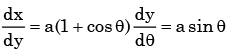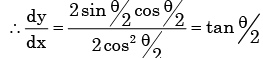Test: Partial Derivatives, Gradient- 2 - Question 5

The minimum value of function y = x2 in the interval [1, 5] is

Detailed Solution for Test: Partial Derivatives, Gradient- 2 - Question 5

y =x 2 is strictly increasing function on [1,5]

∴ y= x 2 has minimum value at x = 1 is 1.

Test: Partial Derivatives, Gradient- 2 - Question 6

The function f(x) = 2x3 – 3x2 – 36x + 2 has its maxima at

Detailed Solution for Test: Partial Derivatives, Gradient- 2 - Question 6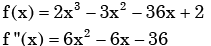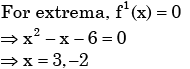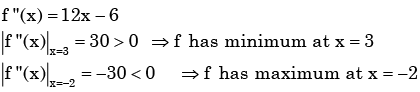Test: Partial Derivatives, Gradient- 2 - Question 7

What should be the value of λ such that the function defined below is continuous at x = π/22?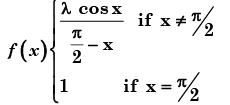Detailed Solution for Test: Partial Derivatives, Gradient- 2 - Question 7

By the given condition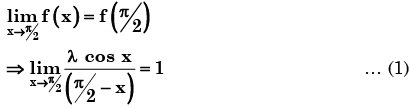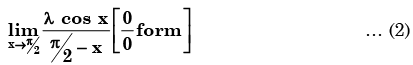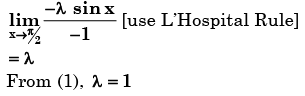Test: Partial Derivatives, Gradient- 2 - Question 8

Consider function f(x) =(x2-4)2 where x is a real number. Then the function has

Detailed Solution for Test: Partial Derivatives, Gradient- 2 - Question 8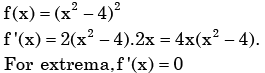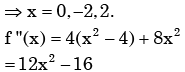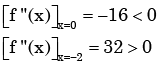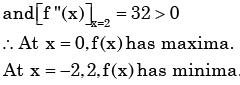Test: Partial Derivatives, Gradient- 2 - Question 9

If f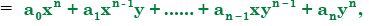where ai (i = 0 to n) are constants, then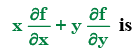Detailed Solution for Test: Partial Derivatives, Gradient- 2 - Question 9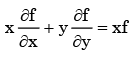- Euler’s theorem for homogeneous function

Test: Partial Derivatives, Gradient- 2 - Question 10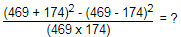Detailed Solution for Test: Partial Derivatives, Gradient- 2 - Question 10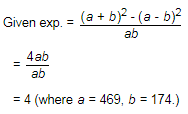Test: Partial Derivatives, Gradient- 2 - Question 11

A point on a curve is said to be an extremum if it is a local minimum or a local maximum. The number of distinct exterma for the curve 3x4 – 16x3 – 24x2 + 37 is

Detailed Solution for Test: Partial Derivatives, Gradient- 2 - Question 11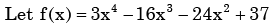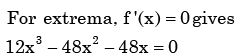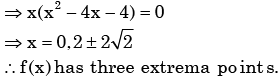Test: Partial Derivatives, Gradient- 2 - Question 12

∇ × ∇ × P, where P is a vector, is equal to

Test: Partial Derivatives, Gradient- 2 - Question 13

The value of the integral of the function g(x, y) = 4x3 + 10y4 along the straight line segment from the point (0, 0) to the point (1, 2) in the x-y plane is

Detailed Solution for Test: Partial Derivatives, Gradient- 2 - Question 13

The equation of the line passing through (0,0) and (1,2)  is y = 2x

Given y x, y ) = 4x3+ 10y4 = 4x3 + 10(2x )4 = 4x3+ 160xy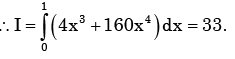Test: Partial Derivatives, Gradient- 2 - Question 14

If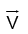is a differentiable vector function and f is a sufficient differentiable scalar function, then curl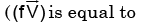Detailed Solution for Test: Partial Derivatives, Gradient- 2 - Question 14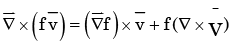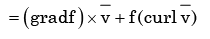Test: Partial Derivatives, Gradient- 2 - Question 15

The temperature field in a body varies according to the equation T(x,y) = x3+4xy. The direction of fastest variation in temperature at the point (1,0) is given by

Detailed Solution for Test: Partial Derivatives, Gradient- 2 - Question 15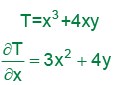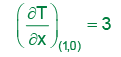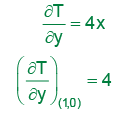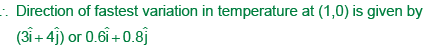Test: Partial Derivatives, Gradient- 2 - Question 16

The divergence of vector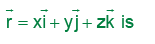Detailed Solution for Test: Partial Derivatives, Gradient- 2 - Question 16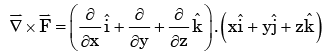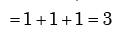Test: Partial Derivatives, Gradient- 2 - Question 17

The divergence of the vector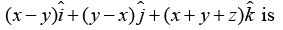Test: Partial Derivatives, Gradient- 2 - Question 18

Among the following, the pair of vectors orthogonal to each other is

Detailed Solution for Test: Partial Derivatives, Gradient- 2 - Question 18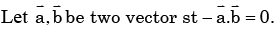Then we say that they are orthogonal.  Choice (c) is correct.

Test: Partial Derivatives, Gradient- 2 - Question 19

The directional derivative of the scalar function f(x, y, z) = x2 + 2y2 + z at the point P = (1,1, 2) in the direction of the vector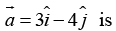Detailed Solution for Test: Partial Derivatives, Gradient- 2 - Question 19

Required directional derivatives at P(1,1,-1)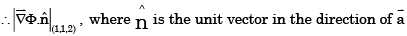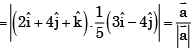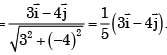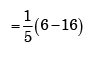=2

Test: Partial Derivatives, Gradient- 2 - Question 20

The Gauss divergence theorem relates certain

Test: Partial Derivatives, Gradient- 2 - Question 21

If P, Q and R are three points having coordinates (3, –2, –1), (1, 3, 4), (2, 1, –2) in XYZ space, then the distance from point P to plane OQR (O being the origin of the coordinate system) is given by

Detailed Solution for Test: Partial Derivatives, Gradient- 2 - Question 21

The equation of the plane OQR is (O being origin).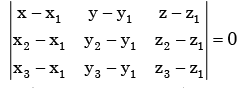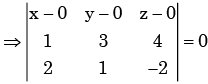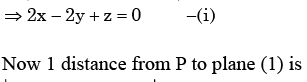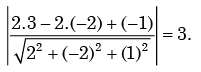Test: Partial Derivatives, Gradient- 2 - Question 22

Let x and y be two vectors in a 3 dimensional space and <x, y> denote their dot product.

Then the determinant det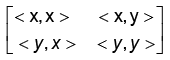Detailed Solution for Test: Partial Derivatives, Gradient- 2 - Question 22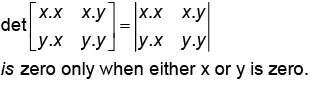Test: Partial Derivatives, Gradient- 2 - Question 23

If a - b = 3 and a2 + b2 = 29, find the value of ab.

Detailed Solution for Test: Partial Derivatives, Gradient- 2 - Question 23

2ab = (a2 + b2) - (a - b)2

= 29 - 9 = 20ab = 10.

Test: Partial Derivatives, Gradient- 2 - Question 24

If a vector R(t)  has a constant magnitude, then

Detailed Solution for Test: Partial Derivatives, Gradient- 2 - Question 24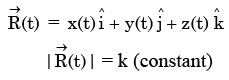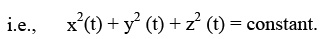On analysing the given (a) option, we find that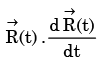will give constant magnitude, so first
differentiation of the integration will be zero.

Test: Partial Derivatives, Gradient- 2 - Question 25

For the scalar field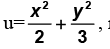magnitude of the gradient at the point(1,3) is

Detailed Solution for Test: Partial Derivatives, Gradient- 2 - Question 25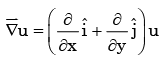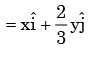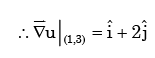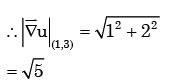## GATE Mechanical (ME) 2023 Mock Test Series

27 docs|243 tests(Scan QR code)